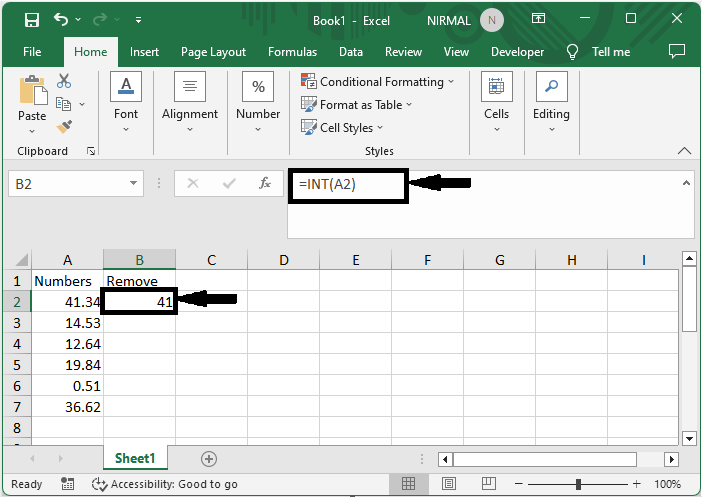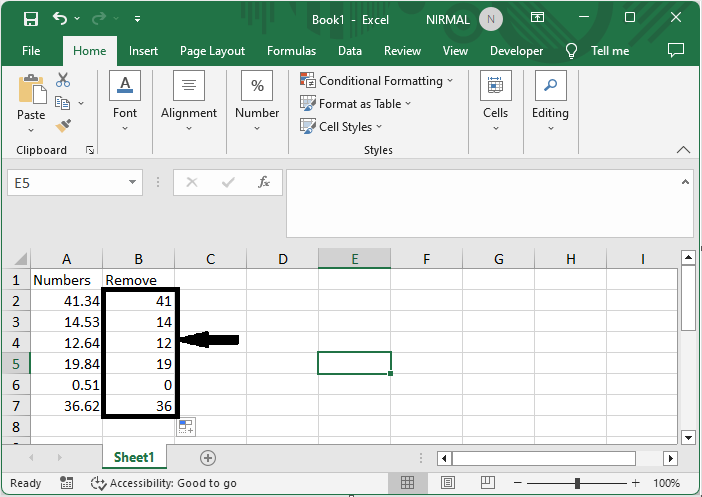# How to Remove Digits After Decimal in Excel?

Numerous functions are available in the powerful spreadsheet programme Excel to manage data, calculations, and formatting. It is commonly required to truncate or eliminate the digits after the decimal point while working with numerical figures, financial data, percentages, or even just to make them easier to understand.

To finish the objective in this tutorial, we will apply the INT formula, which will convert any decimal values to integers while having no effect on the integer values. In this step-by-step manual, we'll examine several approaches to doing this work quickly, taking into account varied scenarios and Excel versions. This article will teach you how to master the methods for removing digits after the decimal, enabling you to work with clear, accurate numerical figures regardless of your level of Excel proficiency.

## Remove Digits After Decimal

Here we will first get any one of the values using the formula, then use the autofill handle to complete the task. So let us see a simple process to know how you can remove digits after decimals in Excel.

### Step 1

Consider an Excel sheet where you have a list of decimal values.

First, click on an empty cell and enter the formula as =INT(A2), then click enter. In the formula, A2 is the cell containing the first value.### Step 2

Then drag down using the autofill handle. Then you will see that decimal points will be removed from the cells.This is how you can remove digits after decimals in Excel.

Note

The formula will convert the decimal numbers to integers by deleting the digits after the decimal.

## Conclusion

In this tutorial, we have used a simple example to demonstrate how you can remove digits after decimals in Excel to highlight a particular set of data.

Updated on: 07-Sep-2023

34 Views